Function Repository Resource:

# LinearlyConsistentQ

Determine if a linear system has a solution

Contributed by: Jordan Hasler, Wolfram|Alpha Math Team
 ResourceFunction["LinearlyConsistentQ"][eqns, vars] compute whether there is at least one solution to the linear system eqns in terms of variables vars. ResourceFunction["LinearlyConsistentQ"][mat] compute whether there is at least one solution to the linear system represented by the augmented matrix mat.

## Details

A system of equations can be written as a matrix-vector equation m.x=b, which can be further written as an augmented matrix.

## Examples

### Basic Examples (1)

Determine if the system of equations has a solution:

 In:=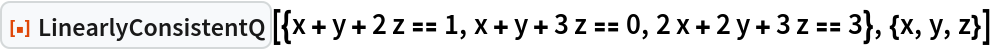Out=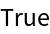### Scope (2)

The system has no solution:

 In:=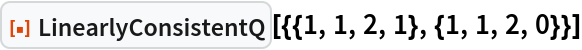Out=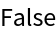Note that the equation cannot be solved:

 In:=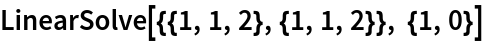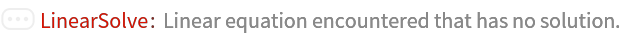Out=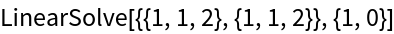The system has a solution:

 In:=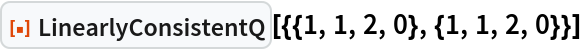Out=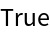Find the solution to the system:

 In:=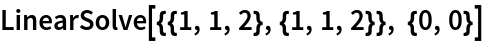Out=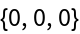## Publisher

Wolfram|Alpha Math Team

## Version History

• 2.0.0 – 23 March 2023
• 1.0.0 – 16 December 2022

## Author Notes

To view the full source code for LinearlyConsistentQ, evaluate the following:

 In:=